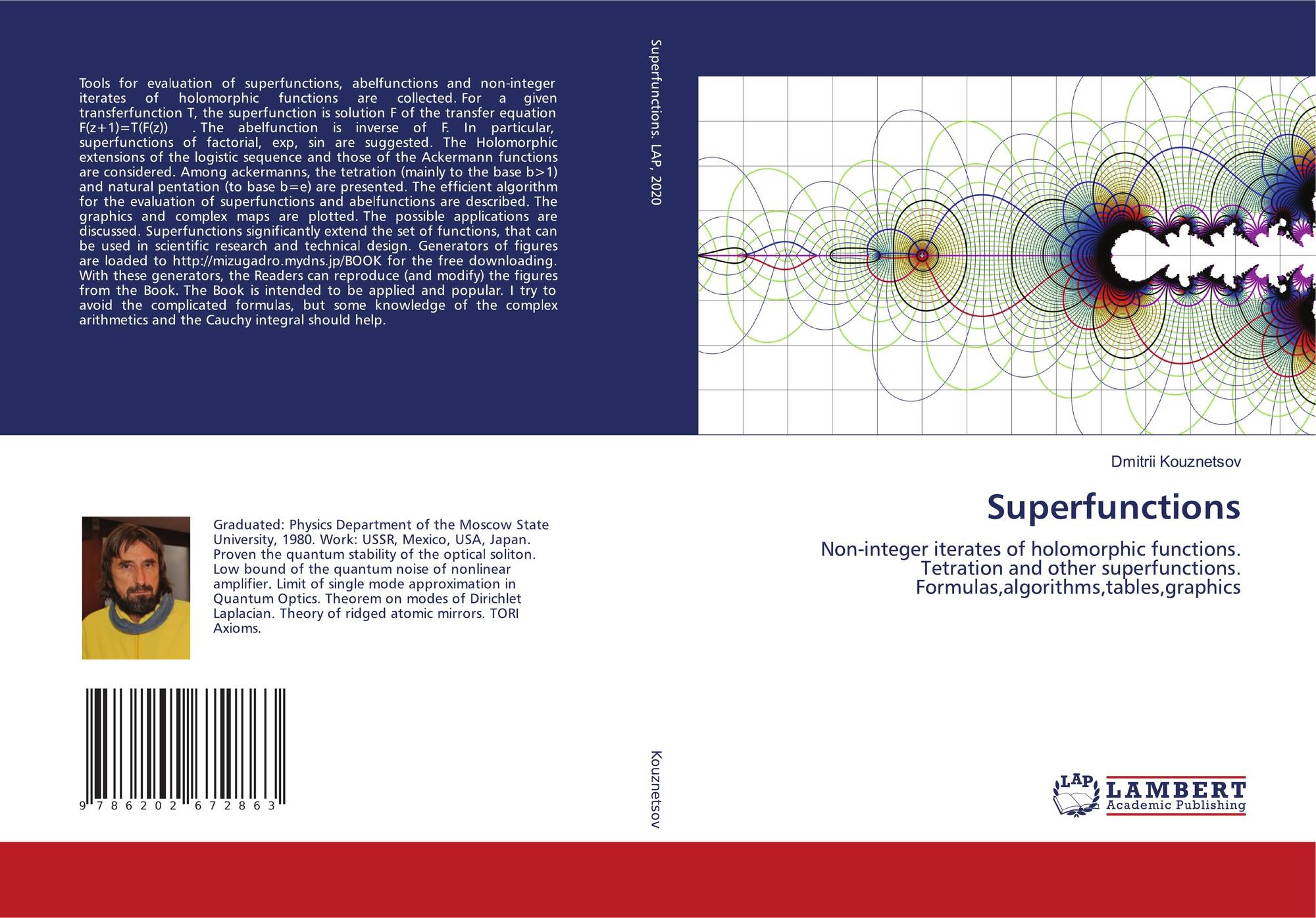## Superfunctions

"Самиздат": [Регистрация] [Найти] [Рейтинги] [Обсуждения] [Новинки] [Обзоры] [Помощь|Техвопросы]
 Ссылки: #author_today_btn{display: block;min-width: 250px;text-align: center;background: #4582af; color: #fff; font-family: Verdana, Sans-serif; padding: 4px 8px; font-size: 14px; font-weight: bold; border-radius: 4px;text-decoration: none;}#author_today_btn:hover{background: #5cb85c;} Конкурсы романов на Author.Today #luk{display:block; text-align:center; background:green; color:#ffffff; font-family: Arial; padding: 4px 10px; font-weight:bold; border-radius:4px; text-decoration:none;} #luk:hover{background:yellow; color:#020234;} Загадка Лукоморья
 Комментарии: 19, последний от 24/08/2020. © Copyright Кузнецов Дмитрий Юрьевич (dima@ils.uec.ac.jp) Размещен: 25/05/2015, изменен: 24/08/2020. 3k. Статистика. Статья: Естествознание Lessons (уроки и методические заметки) Скачать FB2 Ваша оценка: не читать очень плохо плохо посредственно терпимо не читал нормально хорошая книга отличная книга великолепно шедевр Аннотация:For a given holomorphic function T, superfunction F is solution of the transfer equation T(F(z))=F(z+1)"Superfunctions" is book that is published in 2020 by the Lambert Academic Publishing (LAP). You may support the publisher, buying it at
https://www.morebooks.de/store/gb/book/superfunctions/isbn/978-620-2-67286-3

It is free. (Haliava, Sir!)

Below I describe the main topic of the Book.

Assume some given holomorphic function T.
The superfunction is holomorphic solution F of equation
T(F(z))=F(z+1)

The Abel function (or abelfunction) is the inverse of superfunction, G=F-1

The abelfunction is solution of the Abel equation
G(T(z))=G(z)+1

As the superfunction F and the abelfunction G=F-1 are established, the nth iterate of transfer function T can be expressed as follows:
Tn(z)=F(n+G(z))

This expression allows to evaluate the non-integer iterates. The number n of iterate can be real or even complex. In particular, for integer n, the iterates have the common meaning: T-1 is inverse function of T,
T0(z)=z
T1(z)=T(z)
T2(z)=T(T(z))
T3(z)=T(T(T(z)))
and so on. For complex m and n, the group property holds:
Tm(Tn(z))=Tm+n(z)

The book is about evaluation of the superfunction F, the abelfunction G and the non-integer iterates of various transfer function T.

Any critics should be appreciated.
It is difficult to type formulas here (no latex commands seem to be supported), so, I suggest

The Russian version is also available at my site,